# How To Calculate Fixed Cost (With Examples)

Jul. 17, 2022
Articles In Guide
• Formulas

Find a Job You Really Want In

When you manage a business, it’s important to keep track of expenses. Your revenue subtracted by your expenses gives you your net profit, an important measure of how things are going. Your expenses can be broken down into two main categories — fixed cost and variable cost.

Fixed costs are your expenses that are not affected by your business’s sales or production. In other words, fixed costs are independent of business activity and can also be known as overhead or indirect costs.

Variable costs, in contrast, are directly affected by your output. They are the costs incurred for doing business. As the volume of goods or services increases, so will variable costs. Likewise, if the volume of goods or services decreases, the variable costs will decrease.

Key Takeaways:

• Fixed costs can be calculated by adding up all items that are fixed costs or else knowing the total cost and variable cost of each unit produced.

• Fixed costs are periodic expenses that are not affected by output.

• Average fixed cost is the total fixed cost divided by the amount of units produced.

• A break even point for a company can be calculated by dividing the fixed costs by the difference between the price of the unit and the variable cost per unit.

• Marginal cost is the change in cost divided by the change in quantity produced.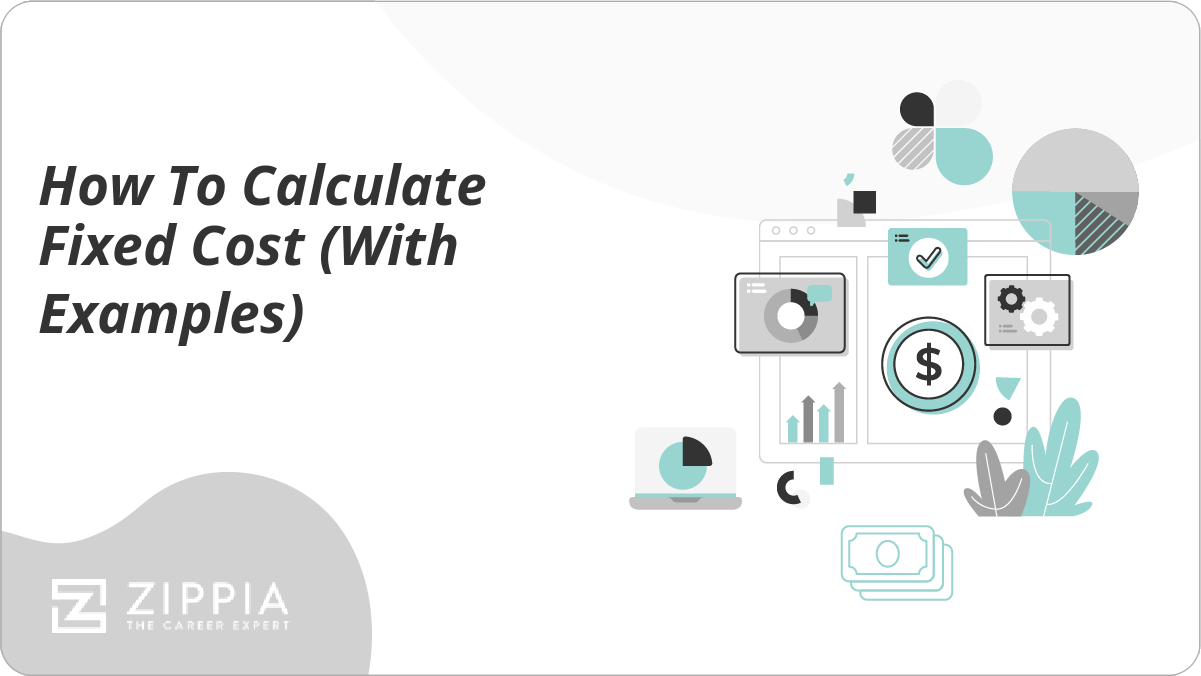## How to Calculate a Fixed Cost Formula

Since fixed costs need to be paid regardless of output production, it is important for a business to accurately calculate its fixed costs. To achieve this, you must use a fixed cost formula.

A fixed cost formula is a formula used in accounting that clarifies which costs are fixed costs within total expenses. There are multiple ways to calculate fixed costs. Below we will examine two different options:

1. Using Multiple Fixed Costs. This works by simply adding all fixed costs together. In this case, you need to have an accurate list of all your costs and know which ones are fixed. The formula can be written as: Total Fixed Cost = F1 + F2 + F3 + …

2. Using Variable Costs. In some cases, businesses only list their total costs and variable costs per unit. You can use this information to determine your fixed costs with the formula: Fixed Cost = Total Cost – (Variable Cost Per Unit * Units Produced).

Each formula has their benefits and drawbacks. Lets take a deeper look at both and use examples to fully understand how they work.

## Fixed Cost Formula: Option 1, Using Multiple Fixed Costs

One way to calculate the total fixed cost is to add up all the expenses that you know are fixed costs. This would be achieved by itemizing all your fixed costs from a list of expenses. The formula would look like this:

Total Fixed Cost = F1 + F2 + F3 + ….

Where Fn is an independent fixed cost.

Let’s break this down in an example:

Imagine you run a small cookie bakery, and you have listed all your costs for the month in an Excel spreadsheet.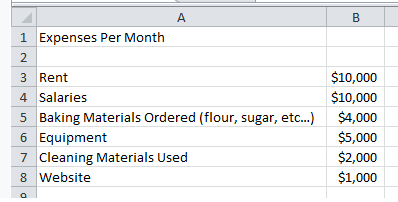First, you need to separate fixed costs from variable costs. In this case, our fixed costs would be rent (B3), salaries (B4), equipment (B5), and website hosting (B8).

Then we would write an Excel formula to add up those costs through the function:
“=B3+B4+B6+B8”.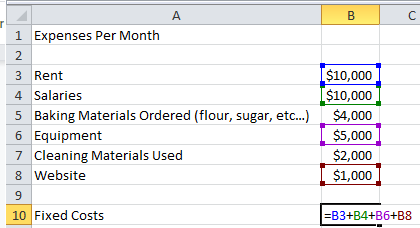When you hit enter, Excel will automatically add up the costs to “\$26,000”.

This option is suitable if your business has a detailed list of expenses. However, there is a downside. You must be able to determine which costs are fixed costs accurately. Also, you must make sure to add up every fixed cost. If this is not possible or too time-consuming, consider the following option to calculate the fixed cost.

## Fixed Cost Formula: Option 2, Using Variable Costs

Some businesses may only list total costs and variable costs per unit. If this is the case, you can use a formula that relies on three factors.

Fixed costs and variable costs add up to create total costs. Therefore, as long as you know your variable cost of production per unit, the number of units produced, and your total production cost, you can calculate the fixed cost.

This fixed cost formula begins by first multiplying the variable cost of production per unit by the number of units produced. Then you take this number and subtract it from the total cost of production. The formula looks like this:

Fixed Cost = Total Cost – (Variable Cost Per Unit * Units Produced)

If we use the cookie bakery example from above:

Imagine that you know your total cost is \$50,000, the variable cost per unit is \$0.60 per cookie, and you have made 40,000 cookies this month. In an Excel spreadsheet, we organized it thus: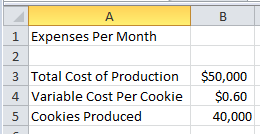Using your formula, you would subtract the total cost of production (B3) by the product of the variable cost per cookie (B4) and the number of cookies produced (B5). In Excel, you will write the formula as =B3-B4*B5. It would look like this: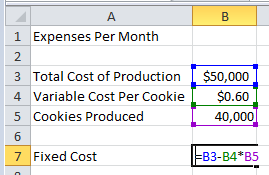When you hit enter, you will see the fixed cost equaling \$26,000, the same amount you calculated with the first formula.

Notice in this formula it is your responsibility to calculate the total variable costs of your business before you determine your fixed cost. It would be reasonable to know your variable cost per unit since this is a cost affected by output. You would also know your output’s total, so your total variable cost becomes a matter of simple multiplication.

## How to Calculate Average Fixed Cost

Once you know the total fixed cost of your business, you can use that information in various ways. For example, the total fixed cost will help with budgeting and pricing.

In particular, if you can calculate the average fixed cost, you will be able to determine the fixed cost per unit. This average fixed cost would be an amount it costs to produce the unit or service, regardless of how many are sold.

Due to this, average fixed cost is beneficial for pricing goods and services. When you know the fixed cost to produce your product or service before you factor in the variable costs, you are able to work with a consistent expense. This consistency helps determine the starting price point of your good or service.

To calculate the average fixed cost, you must take your total fixed cost and divide it by the number of units produced. The formula would like this:

Average Fixed Cost = Total Fixed Cost / Units Produced

You can create an Excel formula for your average fixed costs. All you need to do is take the total fixed cost (B7) and divide it by the number of cookies produced (B5).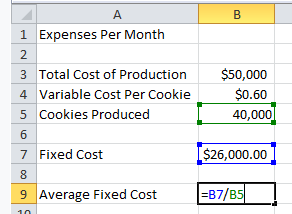Once you hit enter, we will see that your average fixed cost is \$0.65 per cookie.

Now let’s consider what this information would mean for your business. You already know that your variable cost per unit is \$0.60 per cookie. Combine that with your average fixed cost of \$0.65 per cookie, and you have a total cost of \$1.25 per cookie. So if you want to make a profit, you know that your retail sale price will have to be greater than \$1.25 per cookie.

Average fixed cost also shows you how you can increase profit through increased production and sales. The more units you produce and seemingly sell, the fixed cost will be less per unit. If you were to sell 100,000 cookies in a month, your average fixed cost would only be \$0.26 per cookie, which means you could lower the price of your cookies and still increase profit.

This is why large companies that sell high-demand goods and services, such as Walmart, can have low prices while still making a profit. Their average fixed cost per unit decreases significantly due to the size of production output.

## What Is A Fixed Cost?

A fixed cost is a periodic expense that is generally tied to a schedule or contract. A fixed cost is not permanent, but any changes to it will not be directly related to output. This means a fixed cost should be calculated over a certain amount of time, usually a short period of a month, four months, six months, or one year.

Examples of fixed costs include:

• Rent/Mortgage. Wherever your business is located, you will have to pay for the physical location. This cost will not change unless you renegotiate a lease contract or refinance your mortgage.

• Salaries. A salary is a fixed cost of labor. Changes in salaries are not typically related to production output.

• Insurance. Insurance rates, such as property insurance and healthcare costs, will be determined in a contract and calculated as fixed costs.

• Depreciation. Tangible assets such as factory machinery or company vehicles lose their value over time in a predictable manner. An accounting department can calculate this as a cost to the business.

• Taxes. Taxes are costs from federal, state, and local government bodies. Note: some taxes may be related to output, such as taxes on alcohol for liquor stores. But other taxes, such as payroll taxes, must be paid regardless of business activity.

• Interest. Any loan will generate interest that is paid out periodically based on the contract of the loan.

• Fees/Permits. Some businesses pay fees or need permit to conduct operations. These can either be recurring or one time expenses.

## What Is Break-Even Point?

The break-even point is the minimum amount of money a business needs to make to become profitable. In order to find your business’s break-even point, you’ll need to know both your total fixed and variable costs.

Before starting a new business, it’s prudent to come up with at least a projection of your break-even point. And if you’re looking to streamline an already functional business or even a specific project, a break-even point is a good place to start.

The formula for break-even point is:

Fixed costs / (price – variable costs per unit) = Break-even point

Let’s look at an example to flesh this out:

Your business’ total fixed monthly costs (rent, utilities, bills, salaries, taxes) total \$30,000. You sell soft drink products to your region, and the costs of materials and distribution (your variable costs) are \$0.60 and you sell your products for \$2.50.

To find your break-even point, fill in the formula above with the variables:

\$30,000 / (2.5 – .6) = 15,789.5

You’ll have to sell 15,790 soft drinks each month just to break even. Any soft drink you sell after that will net your company a profit.

You can also think of the three categories a business can be in:

• Operating at a loss: Revenue is less than: Variable costs + Fixed costs

• At break-even point: Revenue = Variable costs + fixed costs

• Operating at a profit: Revenue is greater than: Variable costs + fixed costs

## Fixed Cost FAQ

• How do you calculate fixed cost per unit? To calculate fixed cost per unit, start by finding your total fixed costs using one of the methods outlined in this article. Then, divide that number by the total units produced.

For example, if your total fixed costs are \$50,000, and you sold 5,000 units, your fixed cost per unit would be \$10.

• What is the total cost formula? The total cost formula is:

(Average fixed cost X Average variable cost) X number of units produced = total cost

The total cost can provide valuable information about the cost (and therefore viability) of a product line. While total cost can provide a useful metric for simple companies with relatively small product suites, companies that provide a large number of services will find it nearly impossible to precisely determine their total cost.

• How do you find marginal cost? Marginal cost refers to the cost of producing “one more” unit. In other words, if you’re already paying \$5.00 to make 25 cookies, how much more it would cost to make 26 cookies would be your marginal cost.

The formula for marginal cost is:

Marginal cost = change in cost / change in quantity

• What is an example of total fixed cost? Common examples of fixed costs for a business include rent or mortgage, salaries, utility bills, insurance, taxes, and interest. Anything that your business must pay for to remain operational, outside of spending directly on production, is a fixed cost.

Your total fixed cost is simply the result when you add up each individual fixed cost.

Click on a star to rate it!

Average rating / 5. Vote count:

No votes so far! Be the first to rate this post.

Articles In Guide
Never miss an opportunity that’s right for you.Author

Conor McMahon is a writer for Zippia, with previous experience in the nonprofit, customer service and technical support industries. He has a degree in Music Industry from Northeastern University and in his free time he plays guitar with his friends. Conor enjoys creative writing between his work doing professional content creation and technical documentation.

Topics: